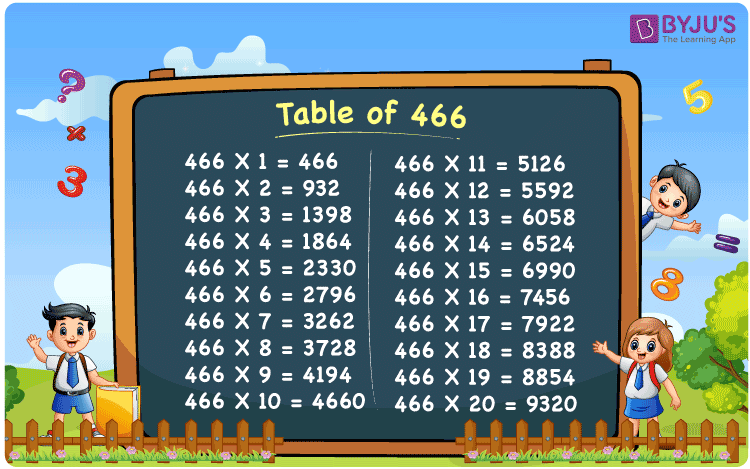Checkout JEE MAINS 2022 Question Paper Analysis : Checkout JEE MAINS 2022 Question Paper Analysis :

# Table of 466

The Table of 466 is provided in a tabular format to help students quickly check the values of multiples of 466. The multiplication table of 466 up to 20 is also available in PDF format to download, which will be handy while solving problems related to the 466 times table. The table of 466 can help do mental maths, which is a vital skill for appearing in any competitive examination.

## Table of 466 Chart

The 466 times table chart up to 20 is given here with an attractive image that can be used as a flashcard to memorise the times table of 466. The Multiplication table of 466 will help students go through the multiples of 466.## What is the 466 Times Table?

The 466 times table is the multiplication of 466 with any natural number. The 466 times table can be obtained by multiplication and by adding 466 repeatedly. The below chart shows the multiplication table of 466, in both multiplication and addition form.

 466×1 = 466 466 458×2 = 916 458 + 458 = 916 458×3 = 1374 458 + 458 + 458 = 1374 458×4 = 1832 458 + 458 + 458 + 458 = 1832 458×5 = 2290 458 + 458 + 458 + 458 + 458 = 2290 458×6 = 2748 458 + 458 + 458 + 458 + 458 + 458 = 2748 458×7 = 3206 458 + 458 + 458 + 458 + 458 + 458 + 458 = 3206 458×8 = 3664 458 + 458 + 458 + 458 + 458 + 458 + 458 + 458 = 3664 458×9 = 4122 458 + 458 + 458 + 458 + 458 + 458 + 458 + 458 + 458 = 4122 458×10 = 4580 458 + 458 + 458 + 458 + 458 + 458 + 458 + 458 + 458 + 458 = 4580

## Multiplication Table of 466

Given below are the first 20 multiples of 466.

 466 × 1 = 466 466 × 2 = 932 466 × 3 = 1398 466 × 4 = 1864 466 × 5 = 2330 466 × 6 = 2796 466 × 7 = 3262 466 × 8 = 3728 466 × 9 = 4194 466 × 10 = 4660 466 × 11 = 5126 466 × 12 = 5592 466 × 13 = 6058 466 × 14 = 6524 466 × 15 = 6990 466 × 16 = 7456 466 × 17 = 7922 466 × 18 = 8388 466 × 19 = 8854 466 × 20 = 9320

## Solved Example on the Table of 466

Example 1:

What is two more than the third multiple of 466?

Solution:

The third multiple of 466 = 466 × 3 = 1398

Two more than 1398 = 1398 + 2 = 1400.

## Frequently Asked Questions on the Table of 466

### What is the times tables of 466?

The 466 times table is the multiplication of 466 with natural numbers. Starting with 466 × 1 = 466, 466 × 2 = 932 , 466 × 3 = 1398 , 466 × 4 = 1864 , 466 × 5 = 2330 and so on.

### What times table have 466?

466 come in the times table of 1, 2, 233 and 466.

### What is 62 times 466?

62 times 466 is 28,892.